# Author an HTML question

An HTML question is actually an extension of the Maple-graded question type, where the input area is replaced with an arbitrary HTML + Javascript application.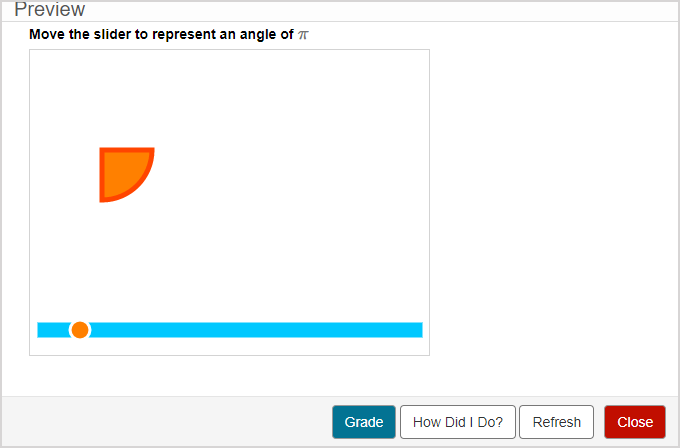You define a correct answer and grading code that use Maple syntax.

The HTML and Javascript that you provide need to take a student’s input and return a representation of their answer to the grading code.

TIP: You can include an algorithm in your HTML question! Check out Author an algorithmic question.

NOTE: The correct answer and algorithmic randomization are hidden from the student and aren't visible by simply inspecting the Javascript on the webpage.

## To author an HTML question

To author an HTML question from the Question Editor:

1. Enter the question statement in the Question Text pane of the Question Editor.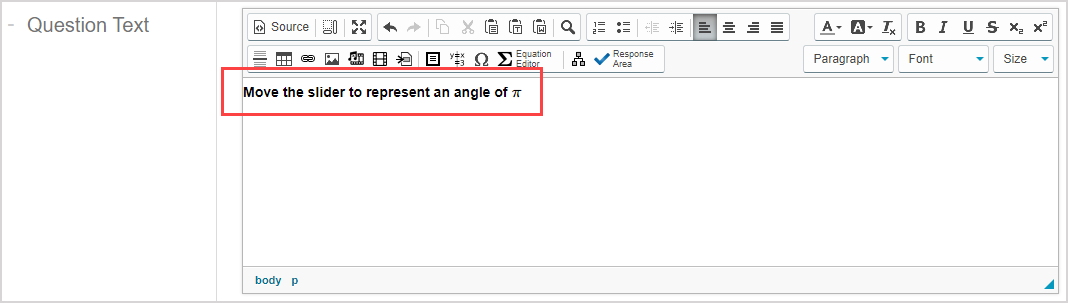TIP: Check out Use the Visual Editor for details on how to use this toolbar to the fullest to control the visual appearance of your HTML question text.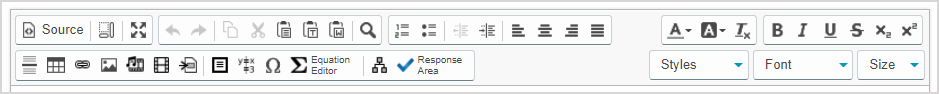1. Click Response Areato select the type of response area for this part of the question.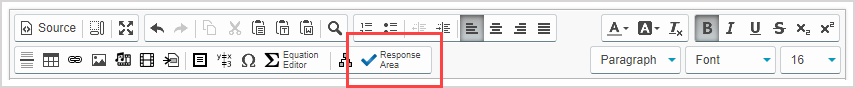NOTE: A question can have multiple question parts. Each question part can have a different type of response area. Example — A question could have the first response area as an HTML response area, and a second response area as multiple choice.

1. Click HTML from the list of response area types.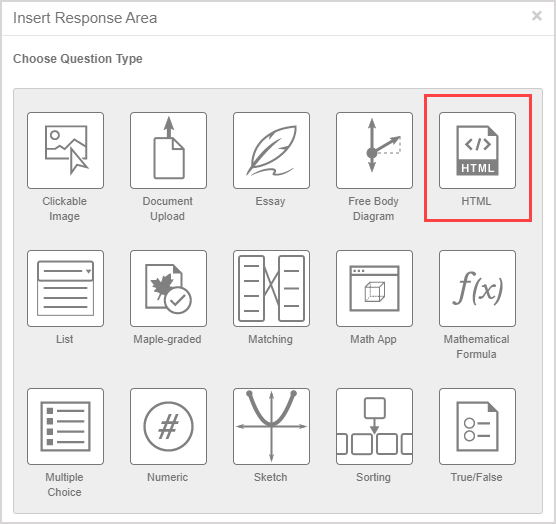1. Assign a weight to the response area. The default response area weighting is 1.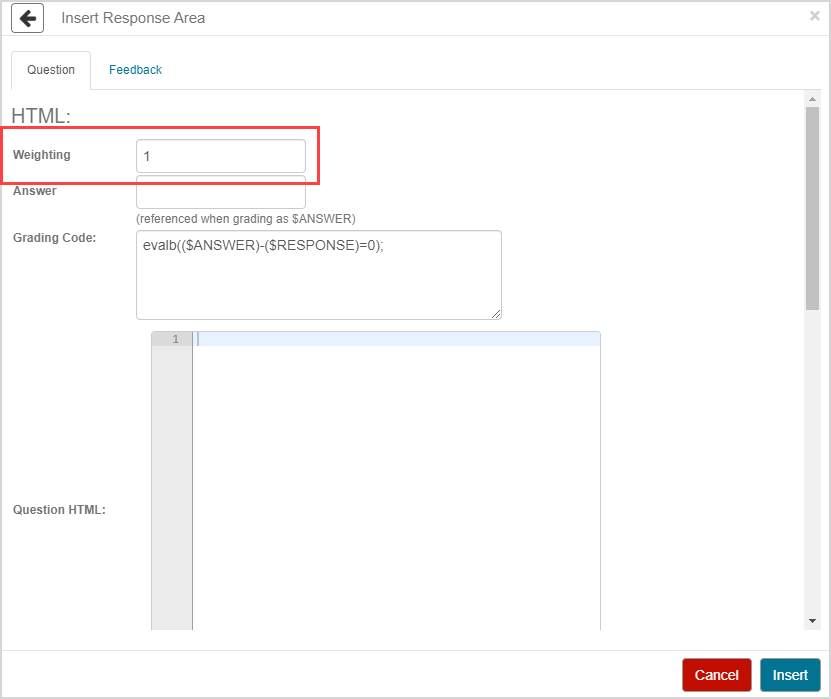TIP: The response area weight can be any integer greater than 0. Response area weighting is proportionate to the question total. Example — A question with two response areas could have the first response area weighted as 2, and the second response area weighted as 4. This means that the second response area will be worth twice as much as the first response area when calculating the total grade for the question.

1. Define the correct response in the Answer field.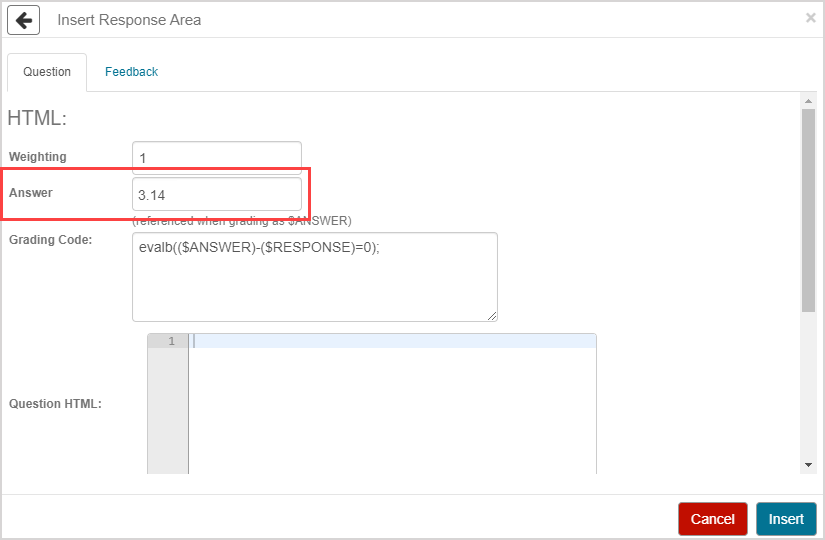TIP: Follow these rules while defining the correct response in the Answer field:

• The last line of code must evaluate to an expression that is stored to the variable \$ANSWER in the grading code, and the Javascript variable answer in the setFeedback() function.
• Complete each line of code in the Answer field with a semicolon (;).
• Add parentheses when appropriate. Example(x + y) / 2
• Use the long form name for all package functions. ExampleVectorCalculus[ArcLength]
• Like a Maple-graded question, you must use valid Maple code to evaluate the answer. Example — Enter 3*x instead of 3x.
1. Define the grading code in the Grading Code field. Exampleevalb( abs(evalf(Pi-(\$RESPONSE))) < 0.4 );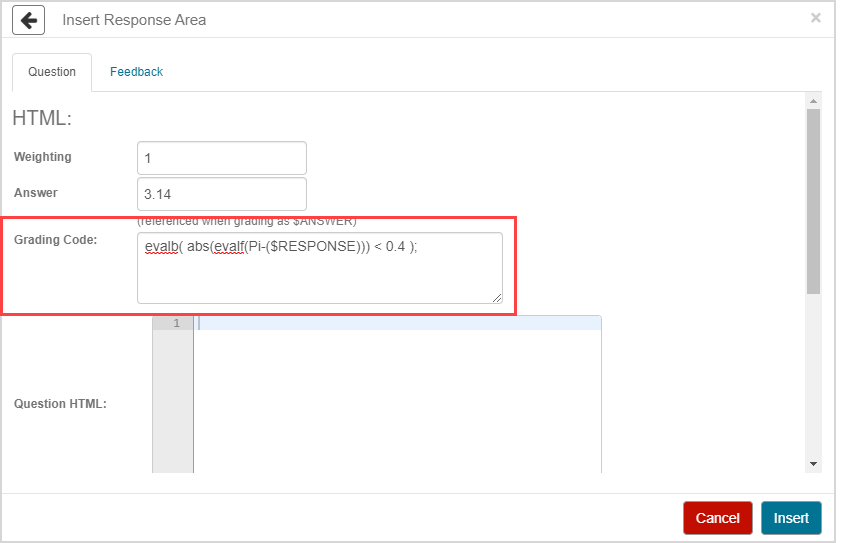TIP: Follow these rules while defining the correct response in the Grading Code field:

• Like a Maple-graded question, you must use valid Maple code to evaluate the answer.
• The last line of your grading code must evaluate to a Boolean value (true or false) or a floating-point number between 0.0 and 1.0 for partial grading.
• By default, use the Maple command evalb((\$ANSWER)-(\$RESPONSE)=0); as indicated, where \$RESPONSE represents the student response and \$ANSWER represents the correct answer.
• Use the long form name for all package functions. ExampleVectorCalculus[ArcLength]
• Use \$RESPONSE to represent the value of the student’s response in your code.
• Before your code is processed by Maple, \$RESPONSE is replaced by the output of the Javascript getResponse() function.
1. Define the HTML needed to display the response area using the Question HTML field.

Example

Copy this code
```1
2
3
4
`<!-- For drawing 2D graphics -->  <script src="https://cdnjs.cloudflare.com/ajax/libs/two.js/0.6.0/two.js"></script>  <div id="canvasContainer"></div>````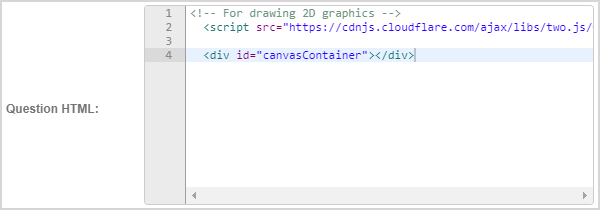NOTE: The entire HTML response area will appear inside of an iframe, so other question text, HTML, and algorithmic variables won't be directly accessible by the question’s Javascript.

TIP: You can add notes to yourself and other authors within your HTML code using the following format:

Copy this code
```1
`<!-- This is a comment within HTML code. -->````
1. Define the CSS needed to display the response area using the Question CSS field.

Example

Copy this code
```1
2
3
`#canvasContainer {    border:1px solid #d3d3d3;}````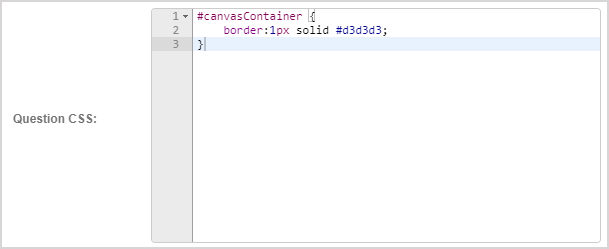NOTE: The response area will appear inside of an iframe, so this CSS won't affect the rest of the question and vice versa.

TIP: You can add notes to yourself and other authors within your CSS code using the following format:

Copy this code
```1
`/* This is a comment within CSS code. */````
1. Complete the initialize(), setFeedback(), and getResponse() functions template in the Question JavaScript field to customize your question.

• initialize() — Called whenever this response area is displayed to either prompt the student for a response or show the student’s response and the correct answer.
• setFeedback(response, answer) — Called when the student’s response and the correct answer are to be displayed next to each other (the variable response will receive the output of getResponse() and the variable answer will receive the value defined in the Answer field).
• getResponse() — Called whenever the question is graded and returns the state of the response area such that it can be evaluated by the grading code.

Example

Copy this code
```1
2
3
4
5
6
7
8
9
10
11
12
13
14
15
16
17
18
19
20
21
22
23
24
25
26
27
28
29
30
31
32
33
34
35
36
37
38
39
40
41
42
43
44
45
46
47
48
49
50
51
52
53
54
55
56
57
58
59
60
61
62
63
64
65
66
67
68
69
70
71
72
73
74
75
76
77
78
79
80
81
82
83
84
85
86
87
88
89
90
91
92
93
94
95
96
97
98
99
100
101
102
103
104
105
106
107
108
109
110
111
112
113
114
115
116
117
118
119
120
121
122
123
124
125
126
127
128
129
130
131
132
133
134
135
136
137
138
139
140
141
142
143
144
145
146
147
148
149
150
151
152
153
154
155
156
157
158
159
160
161
162
163
164
165
166
167
168
169
170
171
172
173
174
175
176
177
178
179
180
181
182
183
184
185
186
187
188
189
190
191
192
193
194
195
196
197
198
199
200
201
202
203
204
205
206
207
208
209
210
211
212
213
214
215
216
217
218
219
220
221
222
223
224
225
226
227
228
229
230
231
232
233
234
235
236
237
238
`function initialize(interactiveMode) {    /*Called when the question is being initialized.    interactiveMode:    if it is true, interaction is allowed on question.    */    /*jQuery.getScript("https://cdnjs.cloudflare.com/ajax/libs/two.js/0.6.0/two.js", function() {*/        let elem = jQuery('#canvasContainer');        /* Run the app */        radians.runApp(elem);    /*})*/    /*Your code starts from here:*/};function setFeedback(response, answer) {    /*called when response or answer is going to     be rendered.    response:     student's response of question.    answer:     correct answer of question.*/    /*Your code starts from here:*/    if (response != "No answer") {        radians.setAngle(response);    }        if (answer != null) {        radians.setAngle(answer);    }};function getResponse() {    /*called when grade button is clicked,    to retrieve back student's response.*/    /*Your code starts from here:*/    return radians.getAngle();};/*Additional functions start from here*//* TwoJS http://jonobr1.github.io/two.js/ *//* colors  orangered #FF8000 rgb(0, 200, 255)*/'use strict';/* Library namespace */let radians = {};(function(rd) {    /* The main application closure */    rd.runApp = function(canvasElem) {        /* app size */        let viewportw = 400;        let viewporth = 300;        /* geometric parameters */        let x0 = 72;        let y0 = 100;        let radius = 50;        let xoffset = 110;        let x;        let y;        let mouse = new Two.Vector();        let styles = {            family: 'proxima-nova, sans-serif',            size: 40,            leading: 40,            weight: 900        };        /* angle constants */        let angle = Math.PI / 2; /* angle in units of pi */        let maxangle = 6 * Math.PI;        /* Make an instance of two and place it on the page.*/        let params = {            width: viewportw,            height: viewporth        };        let two = new Two(params).appendTo(canvasElem);        /* create sliderbar */        let rect = two.makeRectangle(viewportw / 2, viewporth - 20, viewportw - 20, 10);        rect.stroke = 'rgb(0, 200, 255)';        rect.fill = 'rgb(0, 200, 255)'; /* Accepts all valid css color*/        rect.linewidth = 5;        /* create slider marker */        let slidermarker = two.makeCircle(viewportw / 2, viewporth - 20, 10);        slidermarker.fill = '#FF8000';        slidermarker.stroke = 'white'; /* Accepts all valid css color */        slidermarker.linewidth = 3;        /* array to store dynmaic two.js objects */        let elements = [];        rd.drag = function(e) {            let mousex = e.clientX;            if (mousex <= 20) {                angle = 0;            } else if (mousex >= viewportw - 20) {                angle = maxangle;            } else {                angle = maxangle * (mousex - 20) / (viewportw - 40);            }            mouse.set(x, y);        };        rd.dragEnd = function(e) {            jQuery(window)                .unbind('mousemove', radians.drag)                .unbind('mouseup', radians.dragEnd);        };        rd.touchDrag = function(e) {            e.preventDefault();            let touch = e.originalEvent.changedTouches;            radians.drag({                clientX: touch.pageX,                clientY: touch.pageY            });            return false;        };        rd.touchEnd = function(e) {            e.preventDefault();            jQuery(window)                .unbind('touchmove', radians.touchDrag)                .unbind('touchend', radians.touchEnd);            return false;        };        rd.angletox = function(angle) {            return 20 + angle * (viewportw - 40) / maxangle;        };        rd.drawFullCircle = function(x0, y0, r) {            let circle = two.makeCircle(-70, 0, 50);            circle.fill = '#FF8000';            circle.stroke = 'orangered';            circle.linewidth = 5;            return circle;        };        rd.drawWedge = function(ox, oy, ir, or, sa, ea, res) {            let wedge = two.makeArcSegment(ox, oy, ir, or, sa, ea, res);            wedge.fill = '#FF8000';            wedge.stroke = 'orangered';            wedge.linewidth = 5;            return wedge;        };        rd.getAngle = function() {            console.log(angle);            return angle;        };                rd.setAngle = function(a) {            console.log(angle);            angle = a;        };        /* bind mouse/touch evenets */        jQuery(window)            .bind('mousedown', function(e) {                mouse.set(e.clientX, e.clientY);                jQuery(window)                    .bind('mousemove', radians.drag)                    .bind('mouseup', radians.dragEnd);            })            .bind('touchstart', function(e) {                e.preventDefault();                let touch = e.originalEvent.changedTouches;                mouse.set(touch.pageX, touch.pageY);                jQuery(window)                    .bind('touchmove', radians.touchDrag)                    .bind('touchend', radians.touchEnd);                return false;            });        /* This code is called everytime two.update() is called. */        /* Effectively 60 times per second. */        two.bind('update', function(frameCount) {            /* remove previous objects from two */            two.remove(elements);            /* calculates the number of full circles to draw */            let nfullcircles = Math.floor(angle / (2 * Math.PI));            /* draws full circles  */            for (let i = 0; i < nfullcircles; i = i + 1) {                elements.push(radians.drawWedge(x0 + i * xoffset, y0, 0, radius, 0, 2 * Math.PI));            }            /* draws left of radians as a segment */            elements.push(radians.drawWedge(x0 + nfullcircles * xoffset, y0, 0, radius, 0, angle % (2 * Math.PI)));            /* translates the slidermaker to the appropriate x position for the angle */            slidermarker.translation.set(radians.angletox(angle), viewporth - 20);        }).play(); /* Finally, start the animation loop */    }})(radians);````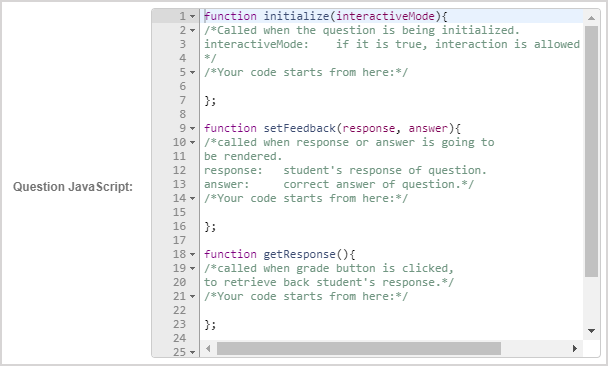TIP: You can add notes to yourself and other authors within your JavaScript code using the following format:

Copy this code
```1
`/* This is a comment within JavaScript code. */````
1. Click Insert to add this response area to the question.1. The response area is inserted into your question.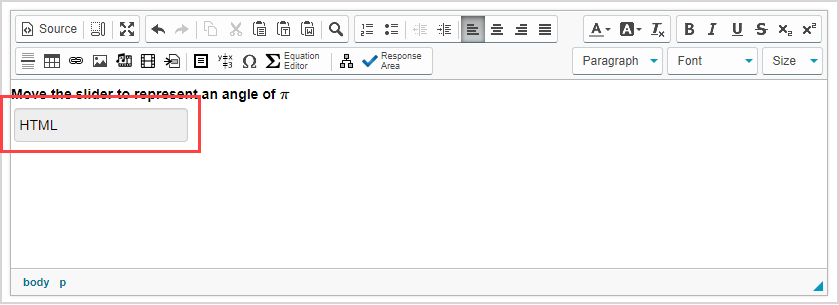1. Click Preview to trial your new question to ensure that it behaves as expected.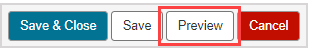TIP: Did you use the example code from this help topic? Here's how your preview will appear:TIP: You first have to click the save iconif you're creating this question from within an Activity Editor. After the question has been saved, you can then click the preview icon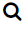to ensure that your new question behaves as expected.

1. Click Save to save your new question. Your new question is now stored in the Questions pane of the Content Repository.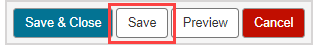TIP: Click the save iconif you're creating this question from within an Activity Editor. This saves your question to the activity and the Content Repository.

## Grading details for HTML questions

HTML questions are graded automatically by Möbius using the grading code that you define.

TIP: If you've created an algorithm in the Algorithm pane of the Question Editor, this algorithm should be referenced by the grading code of your HTML question.